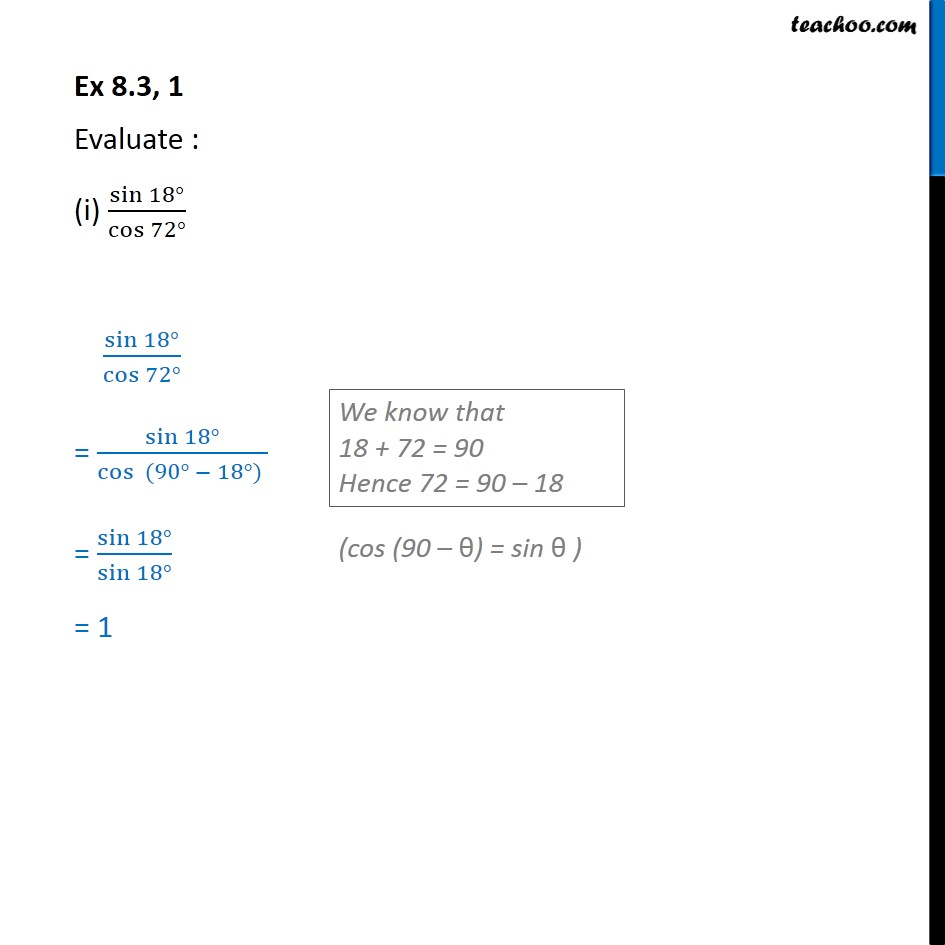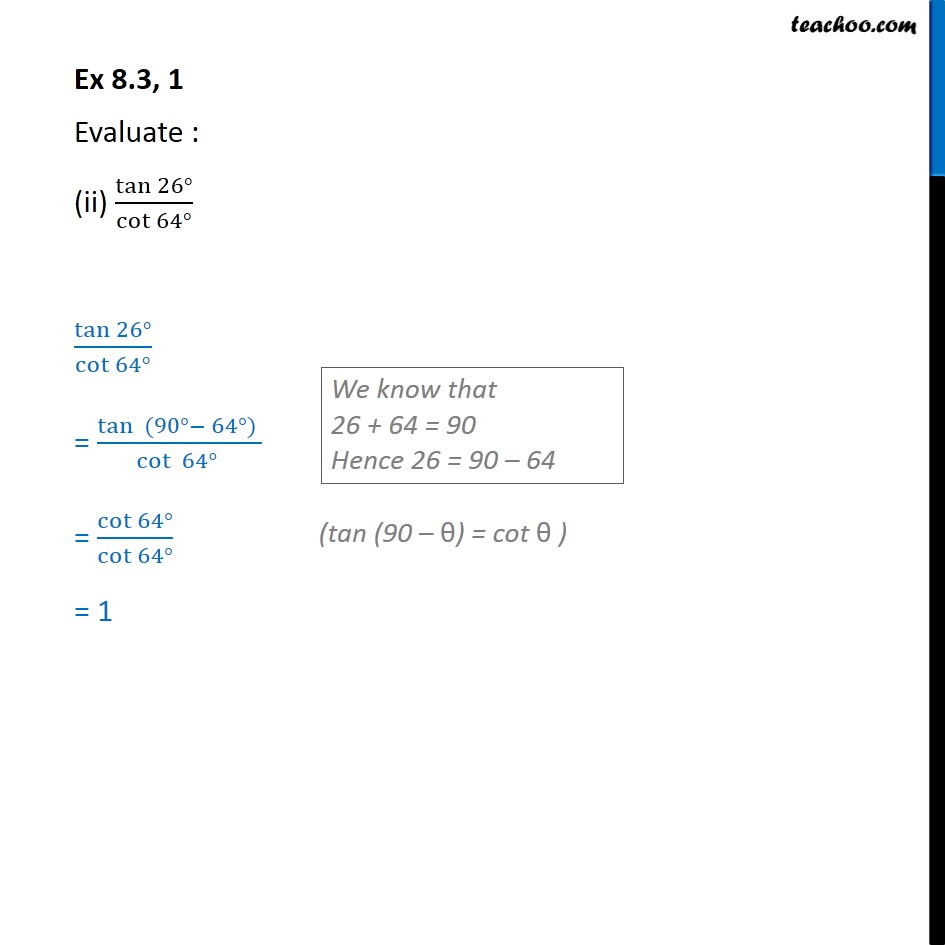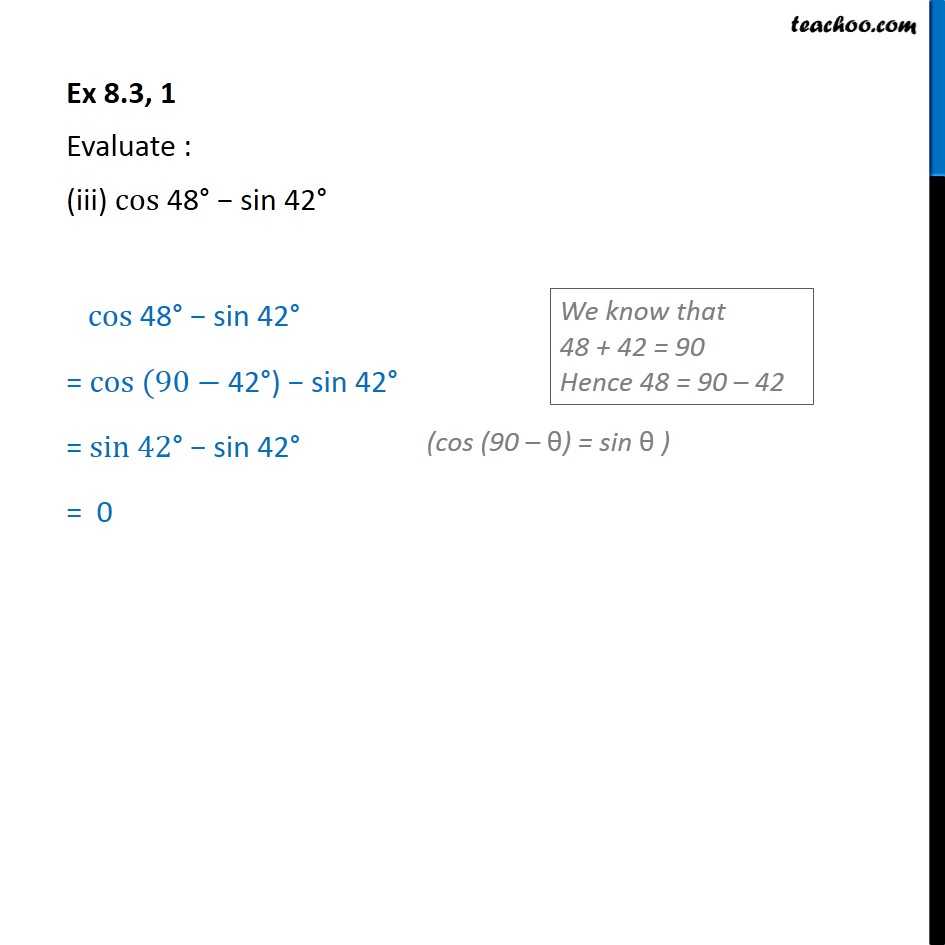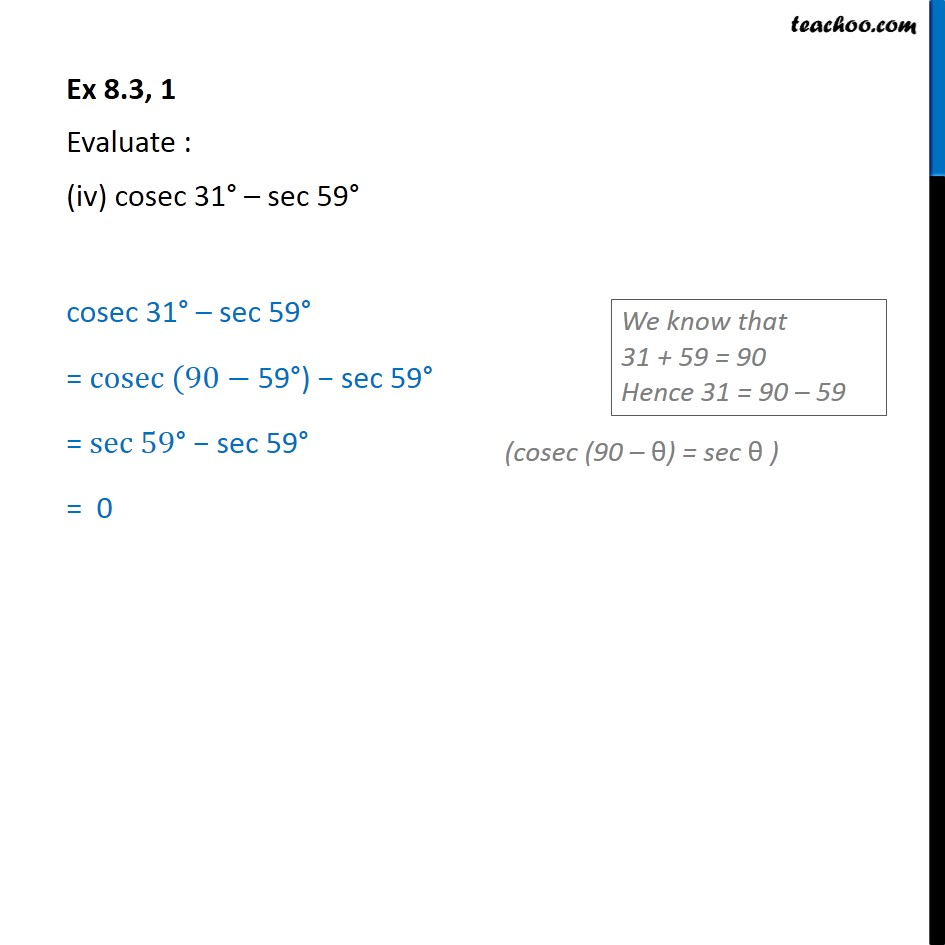Learn all Concepts of Chapter 8 Class 10 (with VIDEOS). Check - Trigonometry - Class 101. Chapter 8 Class 10 Introduction to Trignometry
2. Serial order wise
3. Ex 8.3

Transcript

Ex 8.3, 1 Evaluate : (i) sin 18 /cos 72 sin 18 /cos 72 = sin 18 /cos (90 18 ) = sin 18 /(sin 18 ) = 1 Ex 8.3, 1 Evaluate : (ii) tan 26 /cot 64 tan 26 /cot 64 = tan (90 64 ) /cot 64 = cot 64 /(cot 64 ) = 1 Ex 8.3, 1 Evaluate : (iii) "cos 48 sin 42 " "cos 48 sin 42 " = "cos (90 42 ) sin 42 " = "sin 42 sin 42 " = 0 Ex 8.3, 1 Evaluate : (iv) cosec 31 sec 59 cosec 31 sec 59 = "cosec (90 59 ) sec 59 " = "sec 59 sec 59 " = 0

Ex 8.3